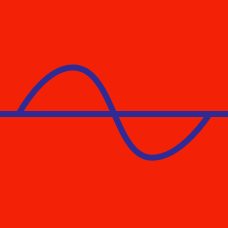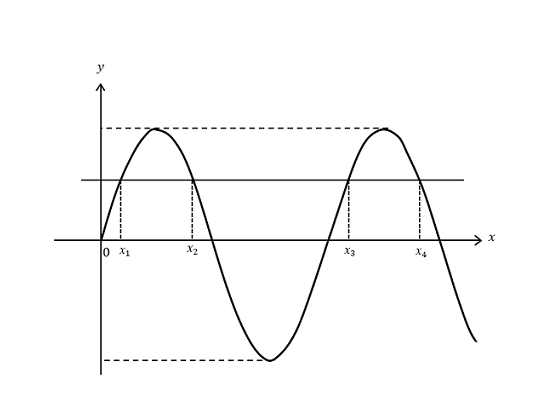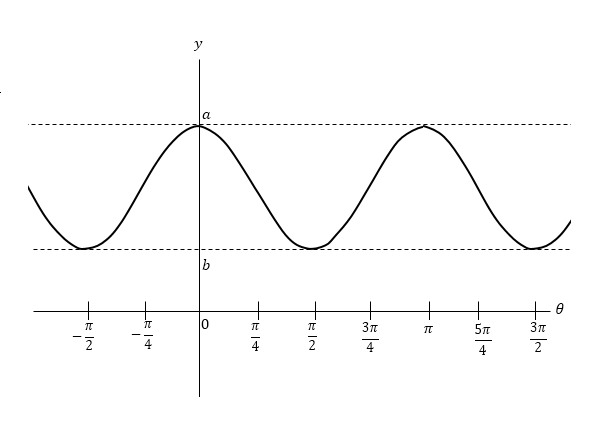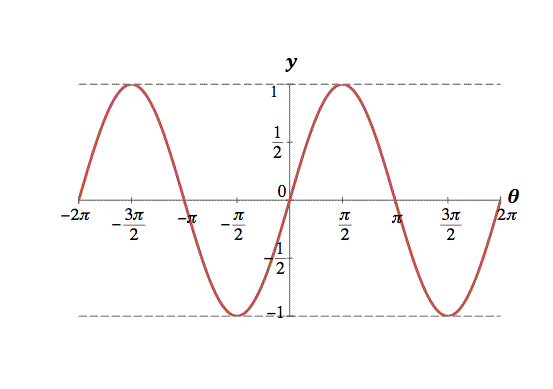Geometry

# Sine and Cosine GraphsThe above curve is the graph of $y=12\sin x$ with $x > 0.$ If $x_{1}, x_{2}, x_{3}$and $x_{4}$ are the $x$-coordinates of the intersection points of the curve and the line $y=6,$ what is $x_{1}+x_{2}+x_{3}+x_{4} ?$

What is the periodicity of the function $y=\sin2\theta ?$The above diagram shows a trigonometric functions with constant period $\pi$. If $a=3$ and $b=1,$ what is the amplitude of the graph ?

Note: the above graph is not drawn to scale.

What is the maximum value of the function $y= 8\sin\theta+11,$ where $0 \leq \theta < 2\pi ?$Which of the following functions corresponds to the above graph?

×